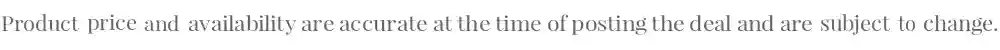captain
10:46 AM - 10, MayPrice: ₹0 MRP: ₹12800 100% Off
DISCUDEMYCOM

What you'll learn

• Introduction to machine learning.
• Linear prediction
• Maximum likelihood and linear prediction
• Ridge, nonlinear regression with basis functions and Cross-validation
• Bayesian learning
• Gaussian processes for nonlinear regression
• Bayesian optimization, Thompson sampling and bandits
• Decision trees
• Random forests
• Spring break
• Random forests applications
• Unconstrained optimization
• Gradient descent and Newton's method
• Logistic regression, IRLS and importance sampling
• Neural networks
• Deep learning
• Importance sampling and MCMC
• Constrained optimization, Lagrangians and duality
• Application to penalized maximum likelihood and Lasso
• Deep Learning
• Reinforcement Learning

### How to get this deal:-

• Click On Get Deal